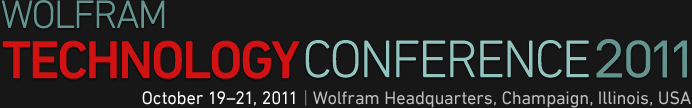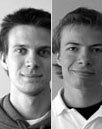# MATHEMATICS & STATISTICS

### Using Mathematica in a Biomedical Statistics Classroom: Blood Glucose Level Analysis

with Iveta Bryjová/Stanislav Hledík
Institute of Physics, Silesian University in Opava, Czech Republic

This contribution demonstrates an example of the possible use of Mathematica in statistics courses for biomedical students. The text, combined seamlessly with statistical computations using built-in features of Mathematica, provides the students a step-by-step guide through statistical analysis pitfalls using a particular case study: blood glucose measurement of a patient suffering from type 1 (juvenile) diabetes mellitus. Both exploratory and inference analysis are included. Attention is particularly paid to the ability of students to select the correct hypothesis test.### Probability & Statistics Overview

with Roger Germundsson

### Interval Computations and Differential Equations

with Petr Girg
University of West Bohemia, Plzeň, Czech Republic

Interval computations are becoming a well-accepted method of rigorous mathematical proofs. We discuss some possibilities of deploying Mathematica into interval-computation proofs of theorems concerning boundary-value problems for strongly nonlinear differential equations. Alternatively, we also describe development of a highly optimized library supporting special functions from the theory of nonlinear differential equations. For performance and correctness reasons, our library is written in GNU Assembler for Intel Pentium. We use new LibraryLink Mathematica 8 technology for calling user-defined C functions. A C interface is used for accessibility of our library functions from Mathematica. In order to improve performance of our library, we derived various approximate formulas for our special functions using symbolic calculations. The library will be used in proving theorems in the theory of nonlinear differential equations.

### Teaching Partial Differential Equations Using Mathematica

with Katarina Jegdic
University of Houston-Downtown

We present an overview of an advanced undergraduate mathematics course on partial differential equations where students are given an opportunity to generate and discover rather than to passively receive knowledge. The course material is motivated through projects that involve "real-world" applications where students use technology to solve various problems. We consider several projects: oil flow recovery, aerodynamics, and heat/wave equations. Each project consists of: (a) derivation of differential equations that describe the particular phenomena, (2) mathematical analysis of the problem and development of a numerical method for approximate solving, (3) implementation of the method using various computer algebra systems, and (4) writing a summary of research findings and/or presenting the findings in the class. Students are given a complete set of notes, consisting of book chapters, research papers, and instructor's notes on mathematical formulation of the problem and development of appropriate numerical methods. The instructor also provides a bone structure of the numerical code that students need to modify, incorporating various partial differential equations and initial conditions.

The proposal on introducing real-world projects and technology in courses on differential equations was developed in the fall of 2007, and its implementation was funded by the Curriculum Development Grant at University of Houston-Downtown in the spring of 2008. The projects were implemented in spring 2009, fall 2009, and spring 2011.### Graph Visualization

with Jae Bum Jung### Numerical Solutions of Differential Equations I

with Rob Knapp### Numerical Solutions of Differential Equations II

with Rob Knapp### Tensor Calculus

with Jose Martin-Garcia/Itai Seggev

### Create Your Own Distribution

with Oleksandr Pavlyk

Using the Mathematica framework to create your own statistics distribution and have it behave just as one of the built-in ones.### Markov Chains

with Oleksandr Pavlyk/Devendra Kapadia### Graph Data Analysis

with Charles Pooh### Introduction to Graphs & Networks

with Charles Pooh

An introduction to the new graph and network functionality.### Reliability Analysis

with Johan Rhodin/Malte Lenzwith Andy Ross

### Quantitative Microbial Risk Assessment for Drinking Water

with Jack Schijven
National Institute of Public Health and the Environment

QMRAspot is a dynamic Mathematica tool that conducts Quantitative Microbial Risk Assessment (QMRA) for drinking water. With one push on a button, microbial data are read from a spreadsheet, distributions are fitted, and a full risk assessment is conducted using Monte Carlo sampling within only a few minutes to yield a risk outcome for drinking water consumption that can be compared with an acceptable health-based target (World Health Organization) or a comparable legislative standard. It is freely available for policy makers, engineers, scientists, and students all over the world.

### Solving Railway Mazes: From Image to Solution

with Antonín Slavík/Stan Wagon
Charles University in Prague, Czech Republic

Roger and Lionel Penrose have created some interesting railway mazes. A railway maze asks the solver to get from start to finish by pretending to be a train that cannot go backward. Thus at every junction the train must follow the curve of the track; it cannot make sharp turns at intersections. We will present a method that starts with an image of such a maze, such as a JPEG file, and finds all solutions. One of the Penrose mazes can be found in the attached notebook. The challenge is to get from A to B by following the curves at each junction.

### Getting the Most from Algebraic Solvers in Mathematica

with Adam Strzebonski

This talk will give a survey of Mathematica functions related to solving algebraic equations and inequalities. It will also discuss the choice of the most appropriate solvers for various types of problems and the ways of formulating the problems so that they can be solved most efficiently.

Download CDF »

### Linear Algebraic Queueing Theory Approach to Reliability

with Mike Ulrey
Boeing

We utilize techniques from a linear algebraic approach to queueing theory to develop a comprehensive set of symbolic reliability formulas, starting from the matrix exponential. Along the way, we also demonstrate some interesting connections to symmetric polynomials and integer partitions and compositions. These methods are quick and easy to illustrate, explain, and use within Mathematica.

*During the conference, not only will you hear about what's new, but you will also be privy to details about what's on the horizon in talks given by Wolfram executives, developers, and more. As such, you will be required to sign a non-disclosure agreement to attend our talks labeled "NDA".